# Scientific Notation Vocabulary Scientific notation a number written

• Slides: 18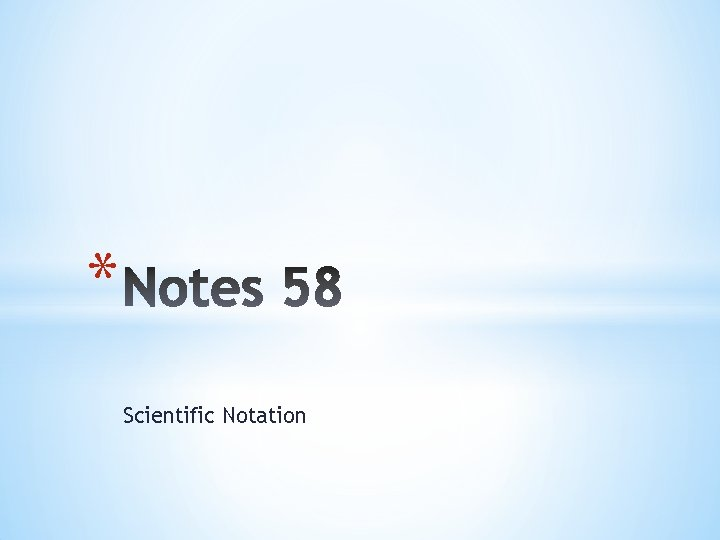* Scientific Notation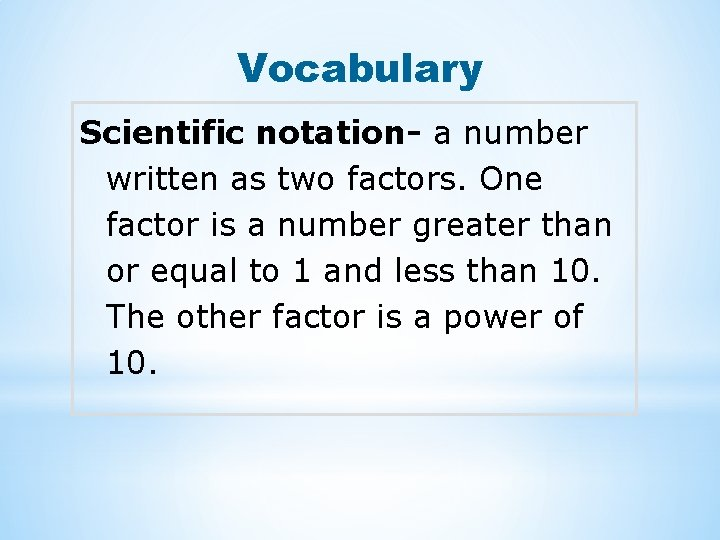Vocabulary Scientific notation- a number written as two factors. One factor is a number greater than or equal to 1 and less than 10. The other factor is a power of 10.An ordinary quarter contains about 97, 700, 000, 000 atoms. The average size of an atom is about 0. 00000003 centimeter across. The length of these numbers in standard notation makes them awkward to work with. Scientific notation is a shorthand way of writing such numbers.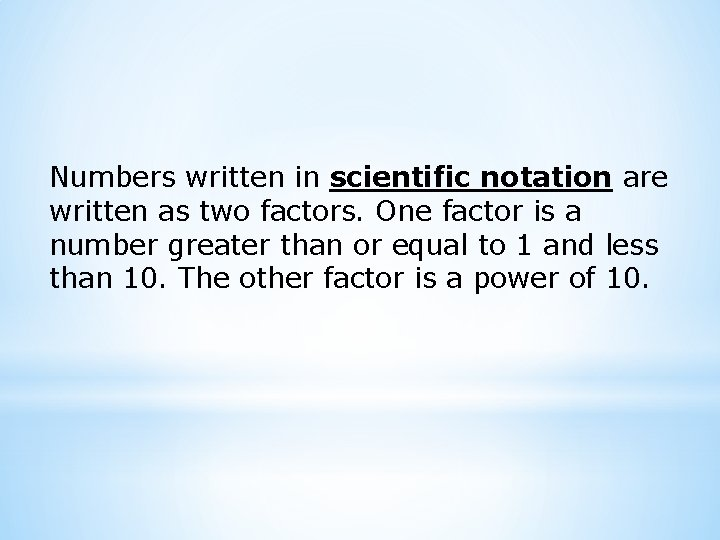Numbers written in scientific notation are written as two factors. One factor is a number greater than or equal to 1 and less than 10. The other factor is a power of 10.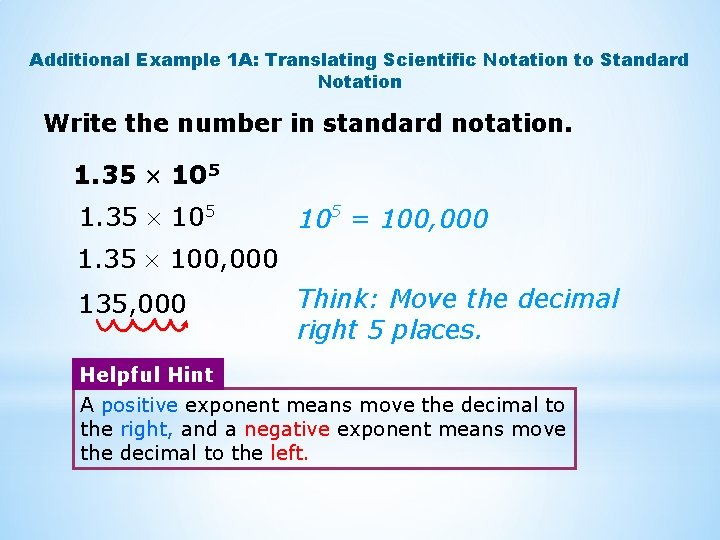Additional Example 1 A: Translating Scientific Notation to Standard Notation Write the number in standard notation. 1. 35 105 1. 35 10 5 105 = 100, 000 1. 35 100, 000 135, 000 Think: Move the decimal right 5 places. Helpful Hint A positive exponent means move the decimal to the right, and a negative exponent means move the decimal to the left.Additional Example 1 B: Translating Scientific Notation to Standard Notation Continued Write the number in standard notation. 2. 7 10– 3 2. 7 10– 3 1 1000 0. 0027 10 – 3 1 = 1000 Divide by the reciprocal. Think: Move the decimal left 3 places.Additional Example 1 C: Translating Scientific Notation to Standard Notation Continued Write the number in standard notation. – 2. 01 104 10 4 = 10, 000 – 2. 01 10, 000 – 20, 100 Think: Move the decimal right 4 places.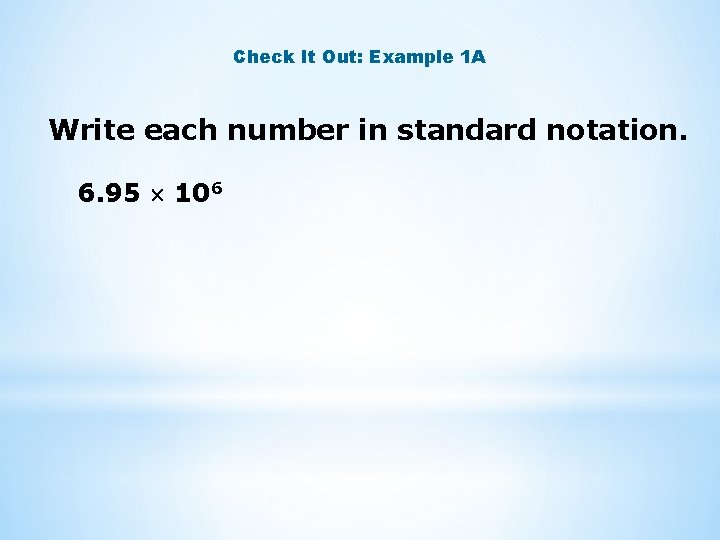Check It Out: Example 1 A Write each number in standard notation. 6. 95 106Check It Out: Example 1 B Write each number in standard notation. 1. 9 10– 8Check It Out: Example 1 C Write each number in standard notation. – 3. 8 10– 5Writing Math To write scientific notation for numbers greater than or equal to 1 and less than 10, use a 0 exponent. 5. 63 = 5. 63 x 10°.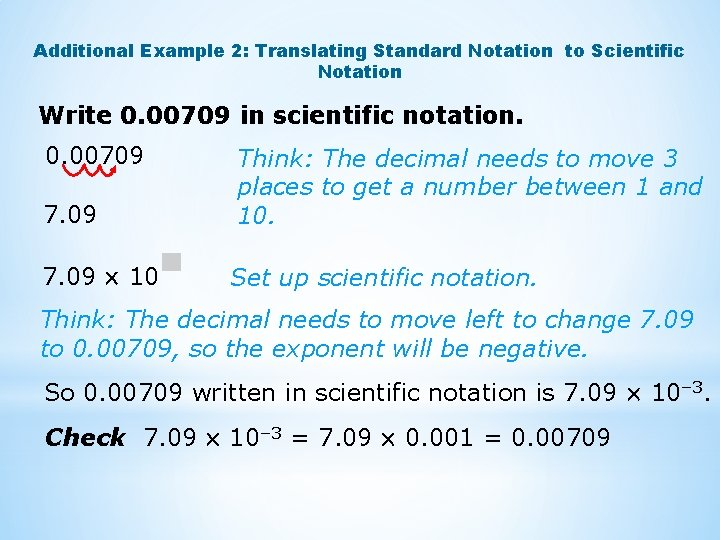Additional Example 2: Translating Standard Notation to Scientific Notation Write 0. 00709 in scientific notation. 0. 00709 7. 09 Think: The decimal needs to move 3 places to get a number between 1 and 10. 7. 09 10 Set up scientific notation. Think: The decimal needs to move left to change 7. 09 to 0. 00709, so the exponent will be negative. So 0. 00709 written in scientific notation is 7. 09 10– 3. Check 7. 09 10– 3 = 7. 09 0. 001 = 0. 00709Check It Out: Example 2 Write 0. 000811 in scientific notation.Additional Example 3: Application A pencil is 18. 7 cm long. If you were to lay 10, 000 pencils end-to-end, how many millimeters long would they be? Write the answer in scientific notation. 1 centimeter = 10 millimeters 18. 7 centimeters = 187 millimeters 187 mm 10, 000 1, 870, 000 mm Multiply by 10. Find the total length. Multiply.Additional Example 3 Continued 1. 87 10 Set up scientific notation. Think: The decimal needs to move right to change 1. 87 to 1, 870, 000, so the exponent will be positive. Think: The decimal needs to move 6 places. In scientific notation the 10, 000 pencils would be 1. 87 106 mm long, laid end-to-end.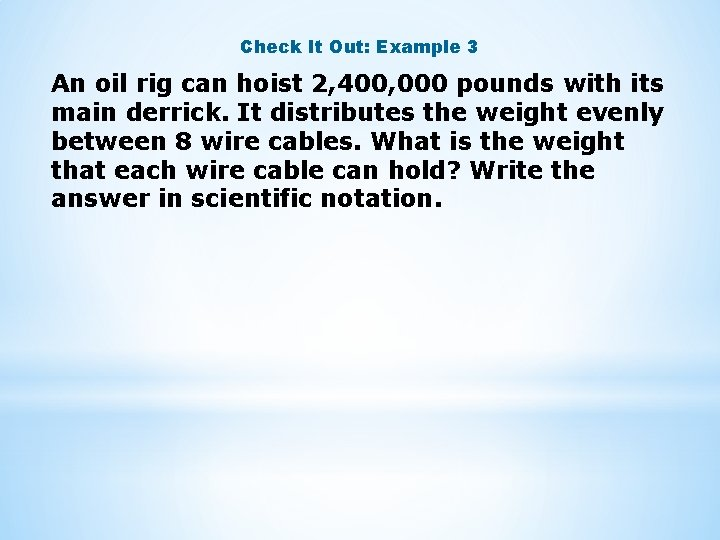Check It Out: Example 3 An oil rig can hoist 2, 400, 000 pounds with its main derrick. It distributes the weight evenly between 8 wire cables. What is the weight that each wire cable can hold? Write the answer in scientific notation.Additional Example 4: Life Science Application One cell has a diameter of approximately 4. 11 x 10 -5 meters. Another cell has a diameter of 1. 5 x 10 -5 meters. Which cell has a greater diameter? 4. 11 x 10 -5 1. 5 x 10 -5 = 10 -5 Compare powers of 10. 4. 11 > 1. 5 Compare the values between 1 and 10. 4. 11 x 10 -5 > 1. 5 x 10 -5 The first cell has a greater diameter.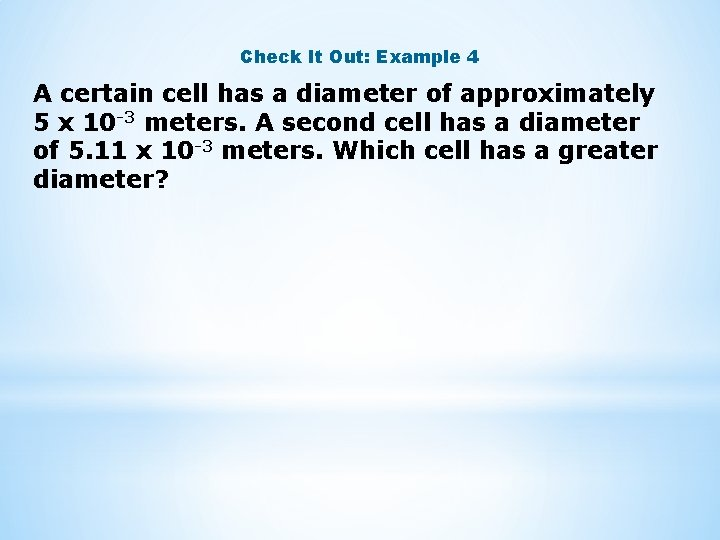Check It Out: Example 4 A certain cell has a diameter of approximately 5 x 10 -3 meters. A second cell has a diameter of 5. 11 x 10 -3 meters. Which cell has a greater diameter?